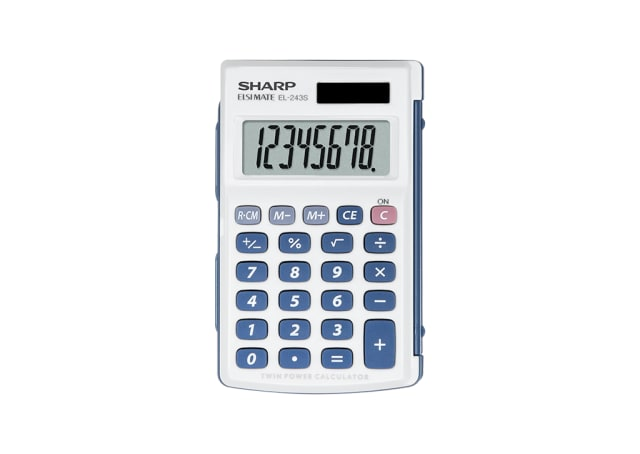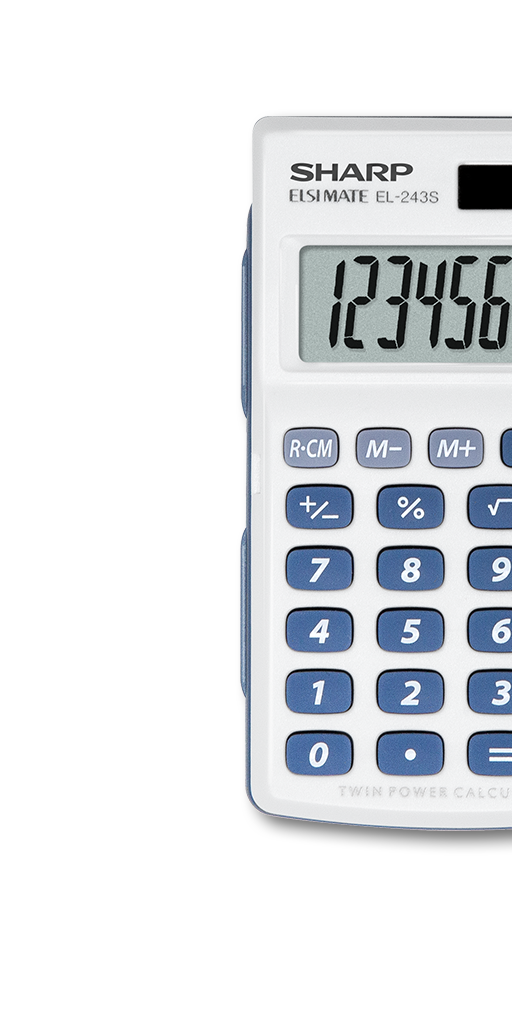EL243S

# 8 Digit Hard Cover Calculator

• 8 digit LCD screen
• Twin power (solar cell & battery LR1130 x 1)
• Large screen display
• Hard cover to protect calculator
• Raised well spaced Keyboard
POA## Pocket Calculator## LCD Display## Twin Powered## Auto Power Off• A compact pocket size calculator for general use, perfect for carrying in pants pockets or small bags for calculating all your daily mathematical equations including percentages.
• LCD display showing up to 8 numbers at a time for convenience viewing.
• Solar Powered with 1x LR1130 alkaline battery, secured by child-safe lock.
• Storing the value of previous equation in memory
• To calculate certain percentage of a number e.g. 100 + 10% gives you 110. Great function for calculating before or after tax amount of a certain item.
• A portion of the equation is automatically memorised as a constant (function Plus, Minus, Division, Multiplication) , thus omitting redundant input. E.g. 2 x 5 = 10, pressing = will multiply by 5 again. This function is great for instances where you'll need to calculate the same equation with a different variable e.g. stocktake or estimating sales
• The calculator powers off automatically when not in use to save battery.
• The square root of a number is a value that when multiplied by itself equals the original number.
Calculator Types
Pocket Calculators
Display
8 Digit LCD display
Dimensions (WxHxD)
64 x 105 x 11 millimetre
Power Source
Solar / LR1130 x 1
Memory
1
Percentage
Yes
Constant
Yes
Square Root
Yes
Auto Power Off
Yes
Key Material
Rubber